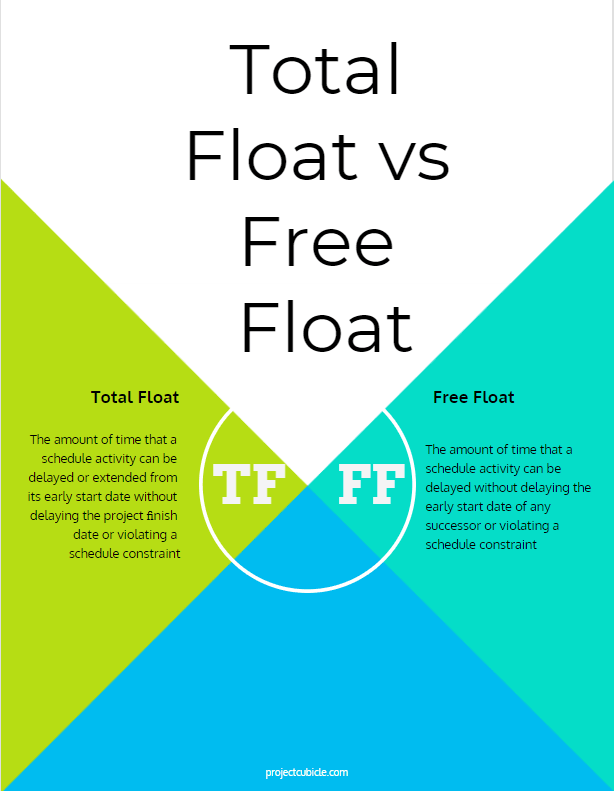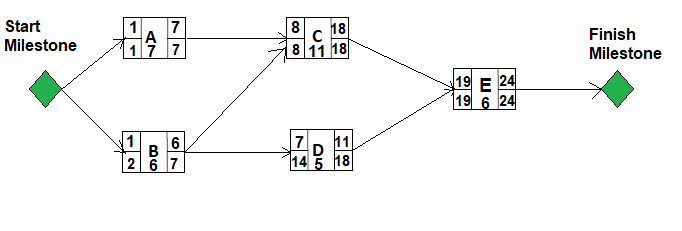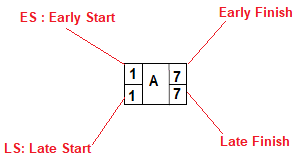## Total Float vs Free Float in Scheduling

2 weeks ago, one of our readers asked how to calculate float time in critical path analysis. Therefore, we decided to write this article. Total Float vs Free Float is one of the most important concepts in PMP Certification training. Basically, TF and FF are two critical path method terms. You use both of them while analyzing the project network diagrams and determining the critical path of a project. Although they sound quite similar, they refer to two different calculation method. In order to analyze project network diagrams, project schedules, and the critical path in the right way, the differences between these two terms should be known. In this article, we will analyze the differences between total float and free float with the help of an example to provide you a better understanding.

Note that they are also used by planning engineers while performing delay analysis and preparing claim files.

Before to start, let’s take a glance at both concepts. Total Float (TF) and  FF definitions are given below.

### Total Float (TF)

While analyzing a project network diagram, schedulers often say “the float of the activity is five days, two days, etc.” But what is the TF and why do we use it?

According to PMI’s  PMBOK Guide, Total Float is “The amount of time that a schedule activity can be delayed or extended from its early start date without delaying the project ﬁnish date or violating a schedule constraint.”

In other words, total float for any activity is defined as the difference between its latest start date and earliest start date.

In a network diagram total float of the critical path is zero.
TF can be calculated by subtracting the Early Start date of activity from its Late Start date or Early Finish date from its Late Finish date.

• TF: LS – ES
• TF: LF – EF

Note that the TF is also known as the slack.

### Free Float (FF)

Free Float is different than Total Float. According to PMBOK Guide, Free Float is “The amount of time that a schedule activity can be delayed without delaying the early start date of any successor or violating a schedule constraint”

Simply we can say that Free Float is the amount of time that an activity can be delayed without delaying the “Early Start” date of its successor. Different than TF which is calculated at the path level, FF is calculated at the activity level.

• Free Float: ES (of successors) – EF of current activity -1

## Total Float and Free Float Example

For better understanding, let’s analyze a simple total float and free float example.

Assume that, we made forward and backward pass calculation and determined the critical path of the below network diagram.The Critical Path is “A-C-E” path because it has the longest duration hence The TF of this path is zero.TF: LS – ES = 1-1 = 0
TF: LF – EF = 7-7 = 0
TF of Activity D = 18-11 =7
TF of Activity B = 7-6=1

#### Which Activities Can Have Free Float ?

Activity B has two successors which are Activity C and Activity D. If there is a delay in Activity B, it delays the activity D, so Activity B has no free float.
Free Float for Activity D
FF: ES (of successors) – EF of current activity -1
FF: ES (of Activity E) – EF of Activity D -1
FF: 19 – 11 -1= 7
Free Float for Activity D is “7”
Activity D can be delayed 7 days without delaying the Early Start date of Activity E.

### Summary

Although, TF and FF sound quite similar, they refer to different concepts. Many people new to project planning and scheduling think that these two concepts are synonymous to each other. Simply put, Total float is the amount of time that an activity can be delayed without delaying the project. On the other hand, free float is the amount of time that an activity can be delayed without delaying the early start of its successor. Simply put, these two floats are widely used to analyze the critical path of a schedule.

Program Evaluation Review Technique